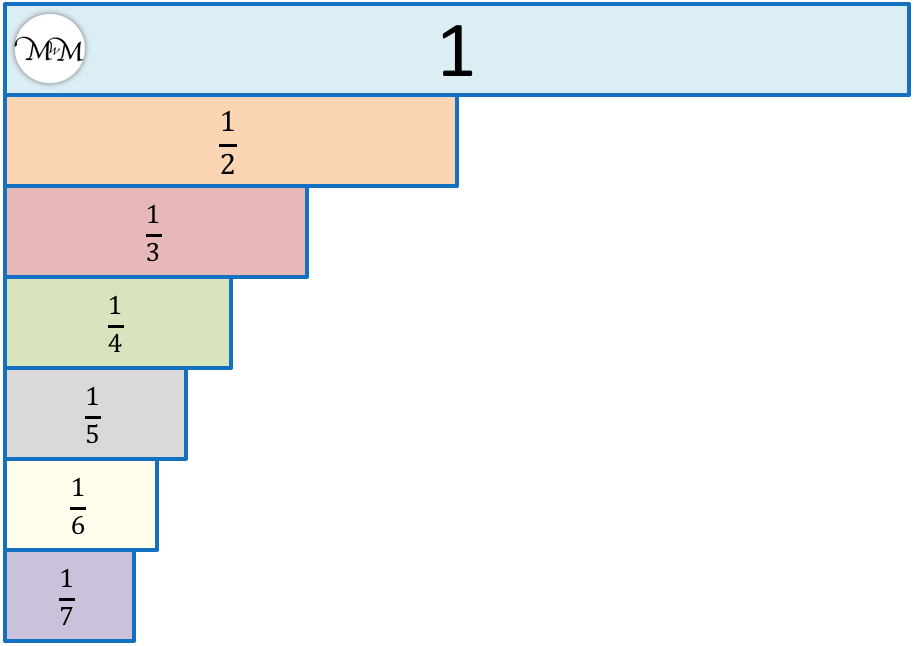# Comparing Fractions with Like Denominators

Comparing Fractions with Like Denominators#### Fraction Wall for Comparing Fractions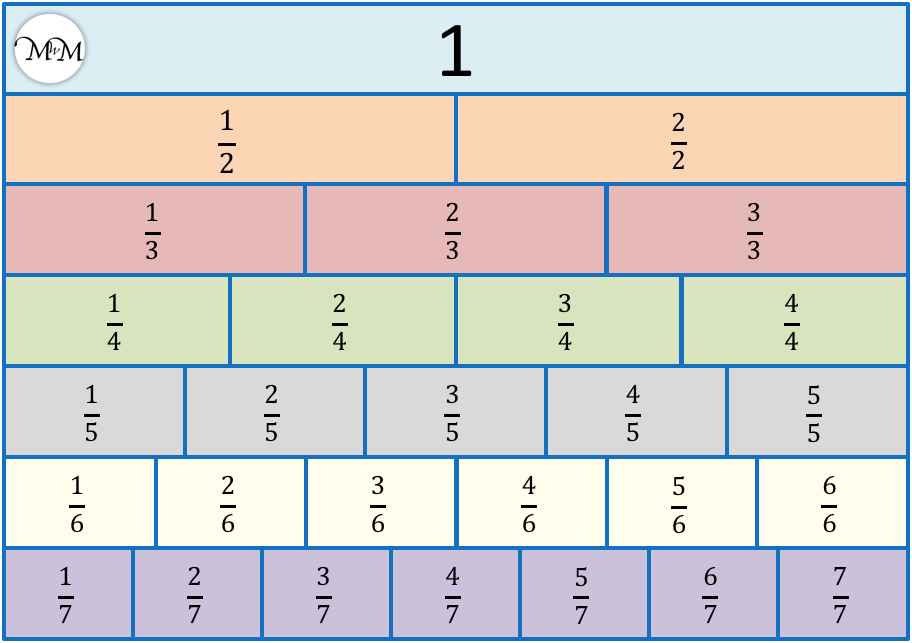• Denominators are the numbers on the bottom of fractions. They tell us how many parts to divide into.
• Numerators are the numbers on the top of fractions. They tell us how many parts we have.
• If fractions have the same denominator then the bigger the numerator, the bigger the fraction.
• Here we have one whole divided into three parts, which are called thirds.
•   3 / 3   is three thirds.
•   2 / 3   is two thirds.
•   1 / 3   is one third.
•   3 / 3   is larger than   2 / 3  , which is larger than   1 / 3  .
• They three fractions have like denominators because they all have a ‘3’ on the bottom.
• The larger the numerator, the larger the fraction.

Fractions with like denominators have the same number on the bottom of the fraction.

The larger the numerator (on top), the larger the fraction.• These fractions have like denominators because they all have a ‘4’ on the bottom.
• We can order fractions with like denominators using the size of their numerator on top.
• We will order these fractions from smallest to largest by writing them in order of their numerators.
•   1 / 4   is smallest because it only has a ‘1’ as its numerator.
•   2 / 4   is next, followed by   3 / 4  .
•   4 / 4   is the largest because it has the largest numerator, which is ‘4’.# Comparing Fractions with Like Denominators

## What are Like Fractions?

Like fractions are fractions that have the same denominator, which means the same number at the bottom of the fraction. Like fractions are also known as similar fractions or are simply called fractions with the same denominator.

If fractions have different denominators then they are unlike fractions.

The denominator is the number at the bottom of a fraction, below the dividing line.

Any set of fractions that have the same number as their denominator are like fractions.

For example, here are like fractions with the same denominator of 3.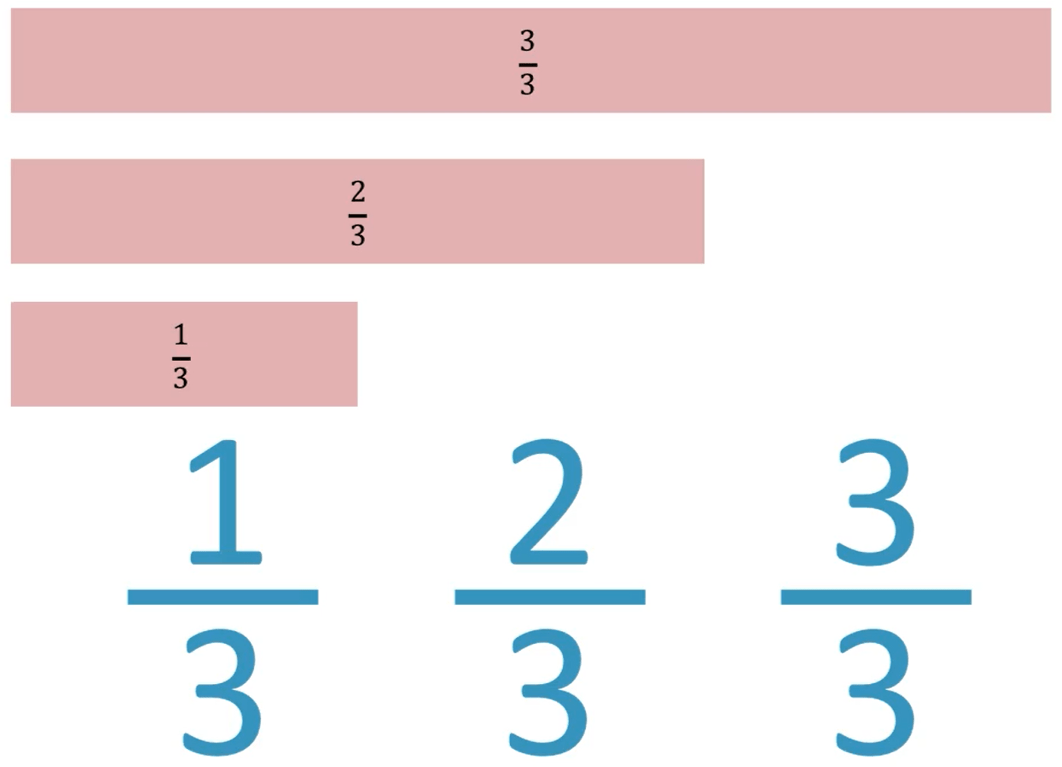We have   1 / 3  ,   2 / 3  and   3 / 3  .

Here is another example of similar fractions with a denominator of 5.We have 2 / 5  ,   3 / 5  and   4 / 5  .

These fractions are like fractions because they all have the same denominator on the bottom of 5.

Here is a fraction wall which shows examples of like fractions. Each row of the wall contains a new set of similar fractions.We can see rows of halves, thirds, quarters, fifths, sixths and sevenths.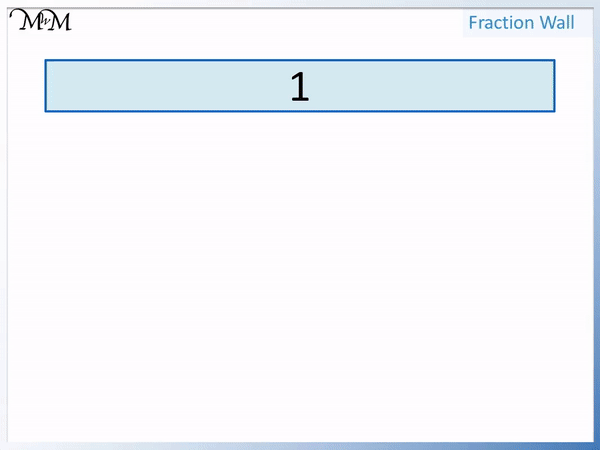## How to Compare Like Fractions

To compare like fractions use the values of the numerators. The larger the numerator, the larger the fraction. The smaller the numerator, the smaller the fraction.

Here is an example of comparing fractions with the same denominator of 3.

We start with one whole and divide it into three equal parts called thirds.Since the denominator is ‘3’, we divide the whole shape into 3 equal parts.

The numerator tells us how many of these parts we have.

The fraction of  3 / 3  has a numerator on top of 3. We have three thirds.

The fraction of  2 / 3  has a numerator on top of 2. We have two thirds.

The fraction of  1 / 3  has a numerator on top of 1. We have one third.We can see that the more parts we have, the larger the fraction we have.

The numerator on top of the fraction tells us how many parts we have. Therefore the larger the numerator, the larger the fraction when comparing like fractions.

3 / 3  has the largest numerator and so is the largest fraction.

1 / 3  has the smallest numerator and so is the smallest fraction.

2 / 3  is in between the other two fractions shown.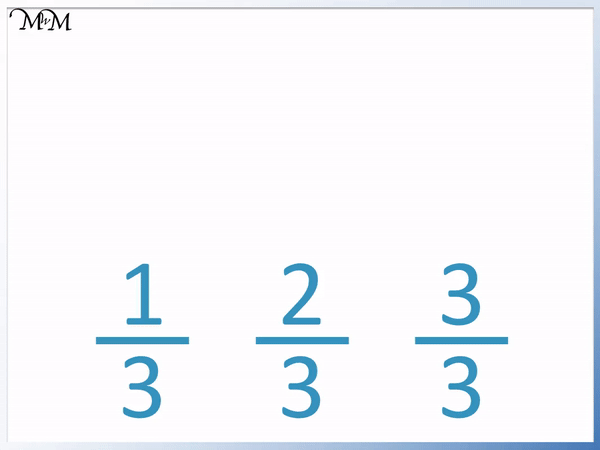Here is another example of comparing like fractions.

We are comparing fractions with the same denominator of 5.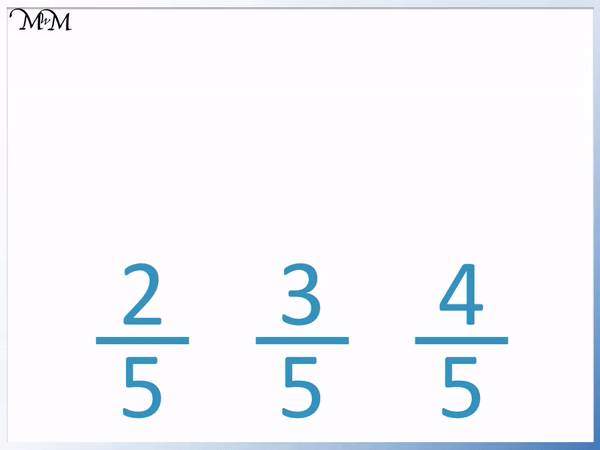To compare like fractions we compare the size of their numerators. The largest fraction has the largest numerator and the smallest fraction has the smallest numerator.

4 / 5  has the largest numerator and so is the largest fraction.

2 / 5  has the smallest numerator and so is the smallest fraction.

The like fractions are put in order from smallest to largest as   2 / 5  ,   3 / 5  and   4 / 5  .## How to Order Fractions with the Same Denominator

To order fractions with the same denominator, order them using their numerator on top of the fraction.

If all of the fractions have the same denominator on the bottom, then simply look at the numerators on top.

In this example of ordering similar fractions we will order them in ascending order.

Ascending order means from smallest to largest.

We have   2 / 4 ,   4 / 4   ,   1 / 4  and   3 / 4  .The fractions are all similar fractions because they all have the same denominator of 4. To put them in order, we put them in order of their numerators on top.

Looking at the numerators alone, the ascending order is 1, 2, 3 and 4.

Therefore the ascending order of these like fractions is   1 / 4 ,   2 / 4  ,   3 / 4 and   4 / 4  .

They are arranged from smallest to largest.

1 / 4  is the smallest fraction and   4 / 4  is the largest fraction.

Here is another example of ordering like fractions from smallest to largest.

We have   3 / 8 ,   7 / 8  ,   5 / 8 and   2 / 8  .

We will arrange the fractions in ascending order from smallest to largest by ordering them using their numerators.Considering only the numerators, we have an order from smallest to largest of 2, 3, 5 and 7.

Therefore the order of the like fractions from smallest to largest is   2 / 8 ,   3 / 8  ,   5 / 8 and   7 / 8  .

2 / 8  is the smallest fraction and   7 / 8  is the largest fraction.Now try our lesson on Comparing Unit Fractions where we learn about unit fractions.